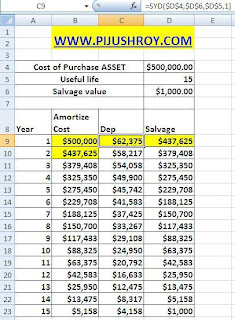### Excel Financial Function to calculate two types Depreciation

MS Excel 2010, 2007, 2003 provides SYD Financial Function to calculate DEPRECIATION under Diminishing Balance Method or Written Down Value Method.

Function :- SYD (cost, salvage, life, per)
Cost :- asset or investment initial cost
Salvage :- value of asset or investment after useful life
Life :- Expected useful life of asset
Per :- for first year 1, second year 2 as so

Path
2007 :- Formula -- Financial -- SYD
2003:- Insert Menu -- Function -- Financial -- SYD -- ok

Question:-
Cost of purchase asset = \$5,00,000.00 (in D4 cell)
Useful life = 15 Years (in D5 cell)
Salvage Value after 15 years= \$1000.00 (in D6 cell)
Per = for 1st year = 1, 2nd year = 2...........1 col - Year
put 1 in A8 cell & 2 in A9 cell. Then Drag it for 15 years i.e. A23 cell

2 col - Amotized Cost: Formula
B9 cell =D4
B10 cell =D9 and Drag formula of B10 cell for 15 years i.e. B23 cell

4 col - Slavage
D9 cell =B9-C9 and Drag it for 15 years i.e. D23 cell

3 col - Depreciation
C9 cell (dep 1st years)
Formula Menu (Excel 2007) -- Financial -- select SYD --

View PictureCost :- asset or investment initial cost
= select cost cell & press F4 for \$\$ sign
Salvage :- value of asset or investment after useful life
= select salvage cell & press F4 for \$\$ sign
Life :- Expected useful life of asset
= select life cell & press F4 for \$\$ sign
Per :- put 1 because 1st year Depreciation is calculated
Then press Ok
Resulted formula =SYD(\$D\$4,\$D\$6,\$D\$5,1)

Drag C9 cell formula to C29 cell & change per value i.e. 1st year 1, 2nd year 2
i.e.
1st year formula =SYD(\$D\$4,\$D\$6,\$D\$5,1)
2nd year formula =SYD(\$D\$4,\$D\$6,\$D\$5,1)

MS Excel 2010, 2007, 2003 provides SLN Financial Function to calculate DEPRECIATION under Straight Line Method

Function :- SYD (cost, salvage, life, per)
Cost :- asset or investment initial cost
Salvage :- value of asset or investment after useful life
Life :- Expected useful life of asset

Path
2007 :- Formula -- Financial -- SLN
2003:- Insert Menu -- Function -- Financial -- SLN -- ok

Question:-
Cost of purchase asset = \$5,00,000.00
Useful life = 15 Years
Salvage Value after 15 years= \$1000.00Follow the Upper Written Down Value Method. Everything are same, only select SLN option instead of SYD option and no PER value .

So formula format is =SLN(\$D\$29,\$D\$31,\$D\$30) instead of =SYD(\$D\$4,\$D\$6,\$D\$5,1)

Check Our New YouTube Video - SUBSCRIBE, LIKE, and SHARE